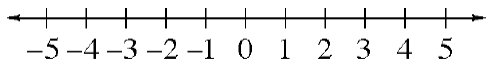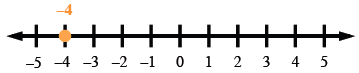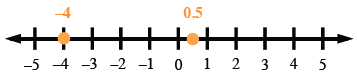Home > CC2MN > Chapter cc21 > Lesson cc21.1.2 > Problem1-14

1-14.

Copy the number line below onto your paper. Place a point on the number line and label the point for each of the following numbers: $-4$, $3$, $0.5$, $-3.5$, $0$, $1$, $-2$, $5$. Homework Help ✎Here is one example.

Here is another example. Can you place and label the rest of the points?